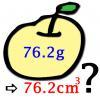#### You may also like### Far Horizon

An observer is on top of a lighthouse. How far from the foot of the lighthouse is the horizon that the observer can see?### Data Matching

Use your skill and judgement to match the sets of random data.### More or Less?

Are these estimates of physical quantities accurate?

# Constantly Changing

##### Age 14 to 16Challenge Level

Physical constants can only be determined by experiment and can never be known exactly, even if in principle an exact value does exists. As a result, physical quantities are given as a probable range of values with an uncertainty registered in the last two digits, as follows:

$1.234\, 5678(32) \rightarrow 1.234\, 5678 \pm 0.000\, 0032$

$245.234\, 789\, 123(45) \rightarrow 245.234\, 789\, 123\pm 0.000\, 000\, 045$

The following table contains the best currently known measurements for various physical quantities:

 Name Value Avogadro constant $6.022\, 141\, 79(30) \times 10 ^{23}$ mol $^{-1}$ Atomic mass constant $1.660 \,538\, 782(83) \times 10^{-27}$ kg Electron mass $9.109\, 382 \,15(45)\times 10^{-31}$ kg Proton-electron mass ratio $1836.152\, 672\, 4718(80)$ Proton mass $1.672\, 621\, 637(83) \times 10^{-27}$ kg Neutron mass $1.674\, 927 \,211(84) \times 10{-27}$ kg Speed of light in vacuum $299\, 792\, 458$ m s$^{-1}$ exactly

Consider the relationship between the error bounds for the proton-electron mass ratio and those for the electron mass and the proton mass. Are they consistent? Which appears to be known to best experimental accuracy?

Using this data, can you work out an upper limit on the mass of a mole of water? What is a lower limit?

How much uncertainty is there is the energy is contained within the mass of a mole of water, according to Einstein's energy-mass equation $E=mc^2$?

If the specific heat capacity of liquid water is about $4.1813$kJ kg$^{-1}$ K$^{-1}$ make an estimate of the number of cups of tea that you could make with this uncertain amount of energy.

NOTES AND BACKGROUND

National Institute of Standards and Technology Reference on Constants, Units and Uncertainty provides detailed information on the bounds of measurements of physical constants.

See http://physics.nist.gov/cuu/Constants/index.html for more details .

For a list of all constants, see http://physics.nist.gov/cuu/Constants/Table/allascii.txt

Interestingly, there is a strong element of statistics used to determine the probable values of constants. Key to this idea are the concepts of error and uncertainty in measurement. Cleverly designed experiments based on a strong understanding of statistics can be used to minimise this uncertainty.